# Basic geometrical ideas

###### Question 1.Use the figure to name: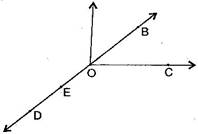(a)Five points

(b)A line

(c)Four rays

(d)Five line segments

(a) Five points are: O, B, C, D, E

(b) A line: DE¯¯¯¯¯¯¯,DE¯,DB¯¯¯¯¯¯¯,DB¯,OE¯¯¯¯¯¯¯,OE¯,OB¯¯¯¯¯¯¯OB¯

(c) Four rays: OD−→−,OD→,OE−→−,OE→,OC−→−,OC→,OB−→−OB→

(d) Four line segments: DE¯¯¯¯¯¯¯,DE¯,OE¯¯¯¯¯¯¯,OE¯,OC¯¯¯¯¯¯¯¯,OC¯,OB¯¯¯¯¯¯¯,OB¯,OD¯¯¯¯¯¯¯¯OD¯

NCERT Solutions for Class 6 Maths Exercise 4.1

###### Question 2.Name the line given in all possible (twelve) ways, choosing only two letters at a time from the four given.###### Question 3.Use the figure to name: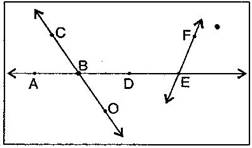(a)Line containing point E.

(b)Line passing through A.

(c)Line on which O lies.

(d)Two pairs of intersecting lines.

Answer: (a) A line containing E = AE¯¯¯¯¯¯¯AE¯ or FE¯¯¯¯¯¯¯FE¯

(b) A line passing through A = AE¯¯¯¯¯¯¯AE¯ or DE¯¯¯¯¯¯¯DE¯

(c) A line on which O lies = CO¯¯¯¯¯¯¯¯CO¯ or OC¯¯¯¯¯¯¯¯OC¯

NCERT Solutions for Class 6 Maths Exercise 4.1

###### Question 4.How many lines can pass though:

(a) one given point?

(b) two given points

Answer: (a) Infinite number of lines can pass through one given point.(b) Only one line can pass through two given points.

###### Question 5.Draw a rough figure and label suitably in each of the following cases:

(a)Point P lies on AB¯¯¯¯¯¯¯.AB¯.

(b) XY¯¯¯¯¯¯¯¯XY¯ and PQ¯¯¯¯¯¯¯PQ¯ intersect at M.

(c) Line ll contains E and F but not D.

(d) OP¯¯¯¯¯¯¯OP¯ and OQ¯¯¯¯¯¯¯¯OQ¯ meet at O.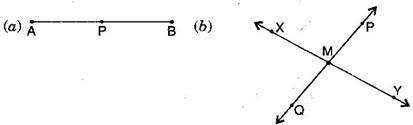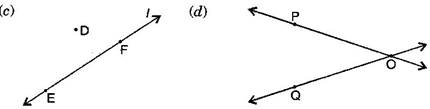NCERT Solutions for Class 6 Maths Exercise 4.1

###### Question 6.Consider the following figure of line MN¯¯¯¯¯¯¯¯MN¯. Say whether following statements are true or false in the context of the given figure: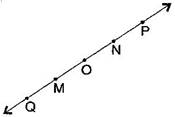(a)Q, M, O, N, P are points on the line MN¯¯¯¯¯¯¯¯MN¯.

(b)M, O, N are points on a line segment MN¯¯¯¯¯¯¯¯MN¯.

(c)M and N are end points of line segment MN¯¯¯¯¯¯¯¯MN¯.

(d)O and N are end points of line segment OP¯¯¯¯¯¯¯OP¯.

(e)M is one of the end points of line segment QO¯¯¯¯¯¯¯¯QO¯.

(f)M is point on ray OP−→−OP→.

(g)Ray OP−→−OP→ is different from ray OP−→−OP→.

(h)Ray OP−→−OP→same as ray OM−→−.OM→..

(i)Ray OM−→−.OM→. is not opposite to ray OP−→−OP→.

(j)O is not an initial point of NP¯¯¯¯¯¯¯NP¯ and NM¯¯¯¯¯¯¯¯NM¯.

Answer: (a) True, (b) True, (c) True, (d) False, (e) False, (f) False, (g) True, (h) False, (i) False, (j) False, (k) True

###### Question 1.Classify the following curves as (i) Open or (ii) Closed.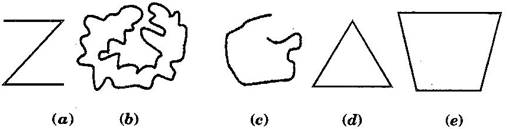(b) Closed curve

(c) Open curve

(d) Closed curve

(e) Closed curve

###### Question 2.Draw rough diagrams to illustrate the following:

(a)Open curve

(b)Closed curve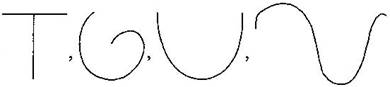Closed curvesNCERT Solutions for Class 6 Maths Exercise 4.2

###### Question 3.Draw any polygon and shade its interior.###### Question 4.Consider the given figure and answer the questions:

(a)Is it a curve?

(b)Is it closed?Answer: (a) Yes, it is a curve.

(b) Yes, it is closed.

NCERT Solutions for Class 6 Maths Exercise 4.2

###### Question 5.Illustrate, if possible, each one of the following with a rough diagram:

(a)A closed curve that is not a polygon.

(b)An open curve made up entirely of line segments.

(c)A polygon with two sides.

Answer: (a)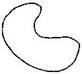(b)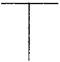(c) Polygon with two sides cannot be draw.

(Ex. 4.3)

Question 1.Name the angles in the given figure: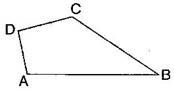Answer: There are four angles in given figure:

ABC, CDA, DAB, DCB

Question 2.In the given diagram, name the point(s):

(a)In the interior of DOE.

(b)In the exterior of EOF.

(c)On EOF.(a) Point interior of DOE : A

(b) Points exterior of EOF : C, A, D

(c) Points on EOF : E, O, B, F

Question 3.Draw rough diagrams of two angles such that they have:

(a)One point in common.

(b)Two points in common.

(c)Three points in common.

(d)Four points in common.

(e)One ray in common.

(a)(b)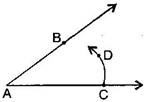(c)(d)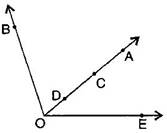(e)(Ex. 4.4)

###### Question 1.Draw a rough sketch of a triangle ABC. Mark a point P in its interior and a point Q in its exterior. Is the point A in its exterior or in its interior?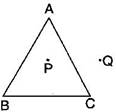A is neither interior of the figure nor exterior of triangle. It is a vertex.

NCERT Solutions for Class 6 Maths Exercise 4.4

###### Question 2.(a) Identify three triangles in the figure:

(b) Write the names of seven angles.

(c) Write the names of sic line segments.

(d) Which two triangles have B as common?(d) Triangles having common B: ΔABC,ΔABC,ΔABD,

###### Question 1.From the figure, identify:(a)The centre of circle.

(c)A diameter.

(d)A chord.

(e)Two points in the interior.

(f)A point in the exterior.

(g)A sector.

(h)A segment.

(a) O is the centre.

(b) Three radii: OA, OB and OC

(c) A diameter: AC

(d) A chord: ED

(e) Interior points: O, P

(f) Exterior point: Q

(g) A sector: OAB

(h) A segment:NCERT Solutions for Class 6 Maths Exercise 4.5

###### Question 2.(a) Is every diameter of a circle also a chord?

(b) Is every chord of a circle also a diameter?

Answer: (a) Yes, every diameter of a circle is also a chord.

(b) No, every chord of a circle is not a diameter.

###### Question 3.Draw any circle and mark:

(a)Its centre.

(c)A diameter.

(d)A sector.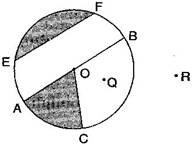NCERT Solutions for Class 6 Maths Exercise 4.5

###### Question 4.Say true or false:

(a)Two diameters of a circle will necessarily intersect.

(b)The centre of a circle is always in its interior.